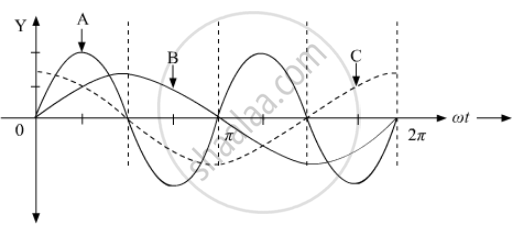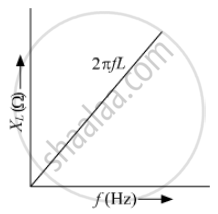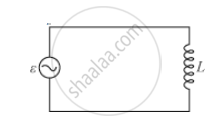PUC Karnataka Science Class 12Department of Pre-University Education, Karnataka
Share

# A device 'X' is connected to an ac source V = V0 sin ωt. The variation of voltage, current and power in one cycle is shown in the following graph - PUC Karnataka Science Class 12 - Physics

#### Question

A device 'X' is connected to an ac source V = V0 sin ωt. The variation of voltage, current and power in one cycle is shown in the following graph:(a) Identify the device 'X'.
(b) Which of the curves A, B and C represent the voltage , current and the power consumed in the circuit? Justify your answer.
(c) How does its impedance vary with frequency of the ac source? Show graphically.
(d) Obtain an expression for the current in the circuit and its phase relation with ac voltage.

#### Solution

(a) The device 'X' is a pure inductor.

(b) Curve A, B and C represents power consumed, voltage and the current in the circuit, respectively. Since, it is given ​V = V0 sin ωt; this sinusoidal variation is represented by the curve B only and in case of inductor, current (I) lags behind the voltage by 90o which is being represented by curve C. Now, we know power is given by
P = V x I

So, the power would be positive for those cycles where both V and I are either positive or negative. Power would be negative when one of the two, voltage or current, is negative. This illustration is followed by only curve A.

(c) Inductive reactance or Inductive impedance, XL = ωL = 2πfL
​This shows that impedance vary linearly with frequency.(d)Source, ε = vm sinωt and let i be the instantaneous current flowing through the circuit.

Using Kirchhoff’s loop rule,

sum epsilon(t)=0

v-(Ldi)/dt=0

(di)/dt=v/L=v_m/Lsinomegat

Integrating di/dt with respect to time,

int (di)/dt dt=v_m/L intsin(omegat)dt

i=-v_m/(omegaL)cos(omegat)+"constant"

because -cos omegat=sin(omegat-pi/2)

therefore i=i_msin(omegat-pi/2)

where, i_m=v_m/(omegaL) is the amplitude of current

Is there an error in this question or solution?

#### APPEARS IN

Solution A device 'X' is connected to an ac source V = V0 sin ωt. The variation of voltage, current and power in one cycle is shown in the following graph Concept: Ac Voltage Applied to a Resistor.
S